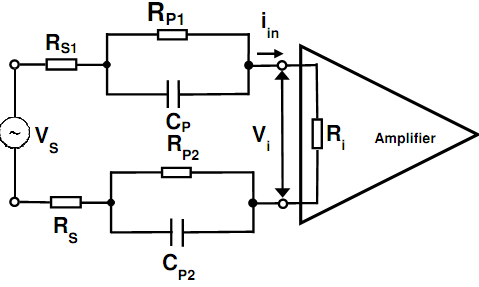Find out the value of phase for values of dc attenuation

For the electrode amplifier interface model outlined within given figure, illustrate that the maximum phase shift introduced within a signal at the amplifier interface happens at a frequency equivalent to the geometric mean of the pole and zero frequencies as √(ωp ωz).Find out the value of given phase for values of dc attenuation of α = 0.5 and 0.02.

#### Related Questions in Electrical Engineering

• ##### Q :Explain about the shared data problem

Explain about the shared data problem.

• ##### Q :Result of excess voltage to CMOS devices

Describe what will happen when excess gate voltage is provided to the CMOS devices?

• ##### Q :What do you mean by op-amp What do you

What do you mean by op-amp? Illustrate briefly.

• ##### Q :Determine the voltages at each of the

Sketch up a table demonstrating the states of conduction of each transistor within the TTL gate demonstrated below for all possible logic combinations of steady-state input states and therefore verify its logical function. Differentiate between reverse and forward mod

• ##### Q :What is multiplexer Explain what is

Explain what is meant by the term multiplexer? Also explain multiplexing displays?

• ##### Q :Transformer draw current Why does a

Why does a practical transformer draw certain current whenever its secondary winding is open?

• ##### Q :Explain clock oscillator circuit and

Explain clock oscillator circuit and clocking Units.

Answer: The Clock is a significant unit of a system. A processor requires a clock oscillator circuit. The clock

• ##### Q :State sampling theorem State briefly

State briefly the term sampling theorem.

• ##### Q :Describe the term AM and FM Describe in

Describe in brief the term AM and FM.